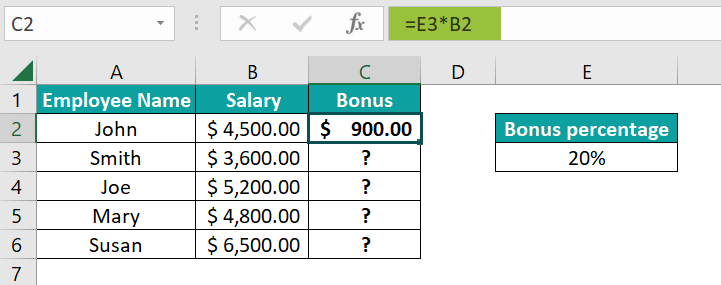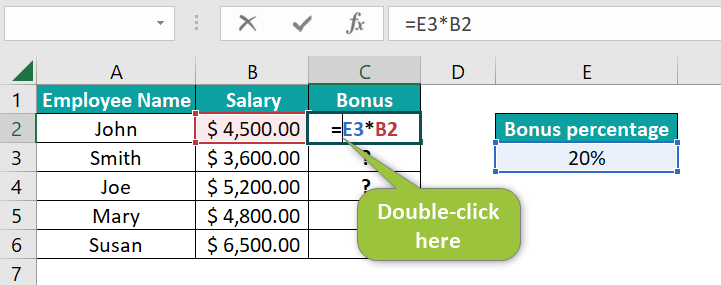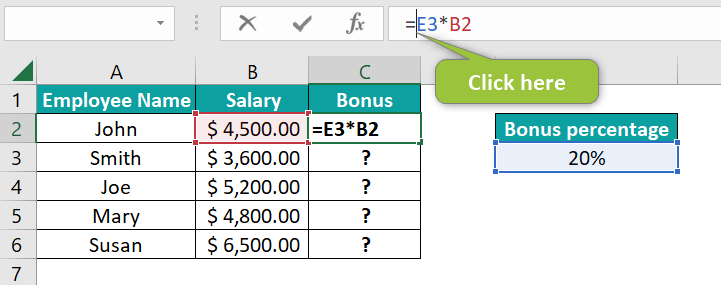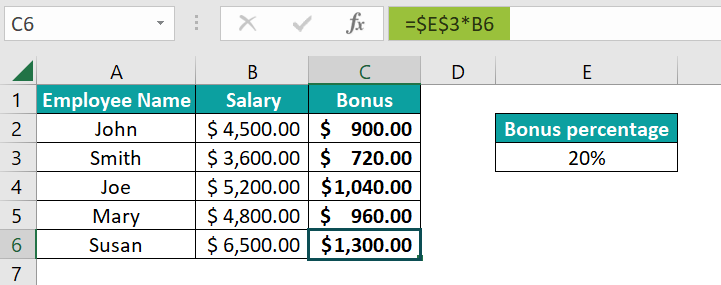# \$ Symbol in ExcelArticle byExcelMojo Team## What is \$ Symbol in Excel?

The \$ symbol in Excel means that the cell references before which we use it are absolute references. In other words, when you use a \$ symbol in an Excel cell, the cell references do not change even when you move the formula to other cells.

For example, enter the formula =A2 * A2 in cell B2. When you drag the Autofill handle to B4, you get the squares of the numbers in Column A. Notice how the references change when we drag the formula from B2 to B4. In cell C2, enter the formula =A2 * \$A\$2. The reference \$A\$2 does not change when you drag the handle. Each number in Column A is multiplied by 3 (the number in reference \$A\$2) to get the result in Column C.

###### Key Takeaways
• The \$ symbol fixes the cell address. Even when a formula is copied or moved, the reference does not change.
• When we add the \$ symbol in front of the column and row header, it becomes an absolute reference.
• When we add the \$ symbol to a row or a column, it is called a mixed reference. For example, \$A1 pr A\$1 is a mixed reference.
• The shortcut to locking a reference in Excel – place the cursor next to the cell reference and press F4.

### Cell References and \$ Symbol

In Excel, formulas usually contain cell references. The references used in the formulas generally change when we copy-paste the formulas to other cells. There are three types of references in Excel.

#### Relative Reference

A cell that contains references without the \$ sign contains relative references. When we copy the formula to another cell, the references get modified.

• Here, we enter =A1 in cell B1 and get the value 25.
• When we copy the formula and paste it into B2, the cell reference automatically modifies to =A2 and displays the value 30.

#### Absolute Reference

When you add the \$ sign before a row and column, the references do not change even when you copy the formula to other cells. So, in absolute reference, it holds the same value it had in the original location.

• We enter a formula =\$A\$1 in cell B1 and get the value 12.
• We enter a formula =\$A\$1 in cell B1 and get the value 12. When we copy the formula to B2, it remains the same due to the \$ symbol, and 12 is displayed again.

#### Mixed Reference

In a mixed reference in excel, we use the \$ symbol only once, either in front of the row number or the column letter. For instance, consider the following:

• \$A1 means Column A does not change while the row changes when we copy the formula
• A\$1 means Column A changes when we copy the formula to other cells while it fixes the row at 1.

### How to Use \$ Symbol in Excel?

The \$ symbol is simple and easy to use. It is helpful when you want the cell references used in any formula to remain the same. One can add the \$ symbol in two ways.

• Insert \$ symbol in Excel manually
• Insert the \$ symbol using a keyboard shortcut, F4

#### Manual Insertion of the \$ Symbol in Excel

Let us look at how we can insert the \$ symbol manually in formulas.

In the example below, the employees of a company are to receive a fixed bonus based on their salaries. Here, we insert the bonus percentage in cell E3.

1. Let us calculate the bonus to be received by each employee. First, multiply the bonus % in cell E3 by each employee’s salary.

Enter the formula =E3*B2 in cell C2.
Press Enter to get the bonus the first employee will receive.2. Now, the formula gets altered if you drag the fill handle or copy-paste it for the next employee in cell C3 because we have given relative references in the formula with no \$ symbol.

To fix the cell reference containing the bonus as an absolute reference, double-click on cell C2 and place the cursor where you want to enter the \$ sign.Alternatively, click the formula bar.Manually enter the \$ symbol in the formula to alter it as =\$E\$3*B2
Press Enter. You get the bonus amount.
When you now copy-paste the formula in cells C2 to C6 for the other employees, the bonus percentage remains fixed because of the absolute reference.#### Inserting \$ Symbol through Keyboard Shortcut F4

Another method of inserting the \$ symbol in Excel shortcut is through the function key F4. Below, we are trying to print the multiplication table for 3.

• Add the formula =A2*D2 to cell B2.
• To make D2 an absolute reference, double-click on cell B2 and select D2, or select D2 in the formula bar.
• Press the F4 key and check the result.
• The \$ symbol gets inserted in the selected part of the formula.

When you press F4 again, the formula changes as seen below and becomes =A2*D\$2.

Press F4 again, and the formula becomes =A2*\$D2, fixing column D.

Thus, using \$ symbol can be done either manually or through the keyboard shortcut F4. Pressing the F4 key again removes all the \$ symbols.

### Examples

We use the \$ symbol in Excel to “fix” a cell address to remain unchanged when we copy-paste the formula across different cells.

#### Example #1

Let us look at an example of how mixed reference can be applied with the \$ sign using the F4 key.

Below is a table containing the price of tickets for an airline for Economy, Business, and First class. The discount percentages are also shared when booking tickets online on three different websites. First, let us look at how mixed reference can be applied here.

Explanation

• Columns C, D, and E should contain the prices of the three classes at discounts of 10%, 20%, and 30%, respectively.
• Rows 3, 4 and 5 should contain the airfares at a discount of 10%, 20%, and 30% for the economy, business, and first-class seats.
• Enter the formula in cell C3 as =C2*B3.
• Now, to fill column C, we need the discount percentage in the second-row cell to be constant(10%) while the cost of the tickets varies. Hence, C2 becomes C\$2.
• Besides, we will use the same column B for calculating the discounts of different air classes. However, their row number changes, Hence B3 becomes \$B3.
• Step 1: Applying these changes to the formula, we get:

As observed, the constant values include Column B and row 2. We obtain the discounts offered by the first website for different class airfares.

• Step 3: Drag the handle horizontally from C3 to E3. You get discounts for economy class at different discount percentages.
• Step 4: Fill in all the other cells by copying the formula. Using mixed references, you get the discount rates by class and for different discount percentages.

#### Example #2

Let us consider another example where we can use absolute and mixed references to calculate the future value of an investment.

Let us consider a simple investment of \$ 5,000 made by an individual. Here, we can calculate its future value at different interest rates over the years.

• Step 1: To calculate the future value, Excel has an in-built financial function FV called the future value excel function. We will use this function to calculate the future value.

In the table below, we have the different interest rates along row 3, while the other payment periods are given along Column A. Enter the FV function in cell B4. Then, we enter the following arguments:

• Rate = rate of interest, cell B3 in this case.
• nper = total payment periods, cell A4 in this case.
• pmt = payment made each period, cell B1 in this case. We write B1 as -B1 since there is a cash outflow from our side, else we will get a negative FV value.

We do not enter the other two optional arguments as this is a simple investment.

• Step 2: Now, we must add the references.
• Since the payment made is fixed, we add absolute references to B1 to lock it. Select B1 and press F4.
• To drag the handle from B4 to B8, we must lock Column A while the numerical row value will change. Now, A4 becomes \$A4.
• When we copy the formula from B4 to F4, row 3 is locked while the column value changes. Hence, B3 becomes B\$3.
• Step 3: Apply the formula to cell B4. You get the initial \$ 5,000 at the end of the first year.
• Step 4: Drag the Autofill handle both vertically and horizontally. You get different future value amounts for various interest rates over different periods.

Notice how the formula changes in cell F8.

Thus, we use the \$ symbol in Excel for locking rows and columns as required.

### Important Things to Note

• By default, references in Excel are relative references, making it easier to copy formulas across and down the cells.
• We can use the \$ symbol in Excel a lot in lookup functions like VLOOKUP, where we have a fixed location for the lookup table.
• Relative references are helpful when using a formula in different cells with varying references.
• Mixed references help you set rows or columns as static based on requirements and can perform complex computations.

1. How to remove \$ symbol in Excel?

When removing the \$ symbol in Excel for absolute or mixed references, select the reference or formula and click on F4. Continue clicking on it till the \$ symbol disappears. If there is no \$ symbol in a formula, it does not mean \$ symbol in Excel not working, but it is a relative reference.

2. Where to find \$ symbol in Excel?

You can insert \$ symbol in Excel either to indicate currency or absolute references. For instance, we can use number formatting to apply the \$ symbol for currency. For references, select the cell reference and click on F4 till you get the \$ symbol as an absolute or mixed reference.

3. Why is \$ symbol in Excel used?

We use the dollar symbol to indicate currency, but it has another vital role in Excel: making a reference an absolute one. Absolute reference means the cell’s address does not change even when the formula is copied across different cells.